# Kiva¶

Kiva is a 2D vector drawing interface providing functionality similar to Quartz, Cairo, the Qt QPainter interface, the 2D drawing routines of OpenGL , the HTML5 Canvas element and many other similar 2D vector drawing APIs. Rather than re-implementing everything, Kiva is a Python interface layer that sits on top of many different back-ends which are in fact provided by some of these libraries, depending on the platform, GUI toolkit, and capabilities of the system.

This approach permits code to be written to the Kiva API, but produce output that could be rendered to a GUI window, an image file, a PDF file, or a number of other possible output formats without any (or at least minimal) changes to the image generation code.

Kiva is the base layer of the Chaco plotting library, and is what is responsible for actually drawing pixels on the screen. Developers interested in writing code that renders new plots or other graphical features for Chaco will need to be at least passingly familiar with the Kiva drawing API.

The most important Kiva backend is the Agg or “Image” backend, which is a Python extension module which wraps the C++ Anti-grain geometry drawing library into a Python extension and exposes the Kiva API. The Agg renders the vector drawing commands into a raster image which can then be saved as a standard image format (such as PNG or JPEG) or copied into a GUI window. The Agg backend should be available on any platform, and should work even if there is no GUI or windowing system available.

## Kiva Concepts¶

This section gives a whirlwind tour of the concepts involved with drawing with Kiva.

### The Graphics Context¶

The heart of the Kiva drawing API is the “graphics context”, frequently abbreviated as `gc` in code. The graphics context holds the current drawing state (such as pen and fill colors, font state, and affine transformations to be applied to points) and provides methods for changing the state and performing drawing actions.

In many common use-cases (such as writing renderers for Chaco), you will be provided a graphics context by other code, but it is straight-forward to create your own graphics context:

```from kiva.image import GraphicsContext

gc = GraphicsContext((400, 400))
```

This is an graphics context for the Agg or “image” backend which has a size of 400x400 pixels. If instead we wanted to draw into a Qt `QPainter` drawing context in a QWidget called my_qwidget we would use:

```from kiva.qpainter import GraphicsContext

gc = GraphicsContext((400, 400), parent=my_qwidget)
```

Other Kiva backends have similar methods of creating a graphics context, and each may take somewhat different arguments to the constructor, depending on the requirements of the backend.

Once you have a graphics context, you can use it to draw vector graphics. For example, the following code will draw a translucent gray line from (100, 100) to (100, 200):

```gc.move_to(100, 100)
gc.line_to(100, 200)
gc.set_stroke_color((0.5, 0.5, 0.5, 0.5))
gc.stroke_path()
```

For many of the backends, you can save the rendered image out as an image file using the `save()` method:

```gc.save("my_line.png")
```

Kiva is numpy-aware, and has a number of methods that allow you to pass numpy arrays of points to draw many things in one operation, with loops being performed in C where possible:

```from numpy import empty, linspace, random

# Nx2 array of points
pts = empty(shape=(20, 2), dtype=float)
pts[:, 0] = linspace(100, 200, 20)
pts[:, 1] = random.uniform(size=20)*100 + 100

gc.lines(pts)
gc.stroke_path()
```

### Coordinate Model¶

Kiva uses mathematical axes direction conventions as opposed to framebuffer axes conventions. In other words, the origin is always at the bottom left of the screen, and the positive y axis goes up from bottom to top; as opposed to screen coordinates which typically have the origin at the top left and the positive y axis goes down from top to bottom.

Additionally, for backends that produce raster images, the coordinates represent the corner of pixels, rather than the center of pixels. This has consequences when rendering thin lines. Compare the two lines in this example, for instance:

```from kiva.image import GraphicsContext

gc = GraphicsContext((200, 100))

gc.move_to(40, 35)
gc.line_to(160, 35)

gc.move_to(40, 65.5)
gc.line_to(160, 65.5)

gc.set_stroke_color((0.0, 0.0, 0.0))
gc.stroke_path()

gc.save("pixel_coordinates.png")
```

Notice that the line on the bottom (the first of the two lines) is fuzzier because it is drawn along the boundary of the pixels, while the other line is drawn through the center of the pixels:### The Coordinate Transform Matrix¶

The Kiva API allows arbitrary affine transforms to be applied to the graphics context during drawing. The API provides convenience methods for common transformations, such as rotation and scaling:

```from numpy import empty, linspace, random, pi
from kiva.image import GraphicsContext

# Nx2 array of points
pts = empty(shape=(20, 2), dtype=float)
pts[:, 0] = linspace(100, 200, 20)
pts[:, 1] = random.uniform(size=20)*100 + 100

gc = GraphicsContext((400, 400))

# draw a simple graph
gc.move_to(100, 200)
gc.line_to(100, 100)
gc.line_to(200, 100)
gc.set_stroke_color((0.5, 0.5, 0.5, 0.5))
gc.stroke_path()

gc.lines(pts)
gc.set_stroke_color((1.0, 0.0, 0.0, 0.5))
gc.stroke_path()

# translate by 100 pixels in the x direction
gc.translate_ctm(100, 0)

# rotate by 45 degrees
gc.rotate_ctm(pi/4.0)

# scale by 1.5 in the x direction
gc.scale_ctm(1.5, 1.0)

# now draw in the transformed coordinates
gc.move_to(100, 200)
gc.line_to(100, 100)
gc.line_to(200, 100)
gc.set_stroke_color((0.5, 0.5, 0.5, 0.5))
gc.stroke_path()

gc.lines(pts)
gc.set_stroke_color((0.0, 0.0, 1.0, 0.5))
gc.stroke_path()

gc.save('transformed_lines.png')
```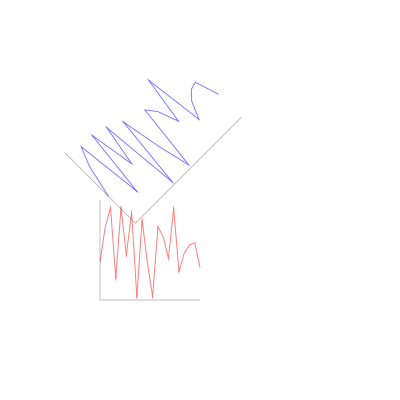If desired, the user can also supply their own transformations directly.

## Paths¶

The basic drawing operations are performed by building a path out of primitive operations, and then performing stroking and/or filling operations with it.

The simplest path operations are `move_to()` and `line_to()` which respectively move the current point in the path to the specified point, and add a line to the path from the current point to the specified point.

In addition to the straight line commands, there are 4 arc commands for adding curves to a path: `curve_to()` which draws a cubic bezier curve, `quad_curve_to()` which draws a quadratic bezier curve, `arc()` which draws a circular arc based on a center and radius, and `arc_to()` which draws a circular arc from one point to another.

Finally, the `rect()` method adds a rectangle to the path.

In addition there are convenience methods `lines()`, `rects()` and `line_set()` which add multiple lines or rectangles to a path, reading from appropriately shaped numpy arrays.

None of these methods make any change to the visible image until the path is either stroked with `stroke_path()` or filled with `fill_path()`. The way these actions are performed depends upon certain state of the graphics context.

For stroking, the graphics context keeps track of the color to use with `set_stroke_color()`, the thickness of the line with `set_line_width()`, the way that lines are joined with `set_line_join()` and `set_miter_limit()`, and the way that they are ended with `set_line_cap()`. Lines can also be dashed using the `set_line_dash()` method which takes a pattern of numbers to use for lengths of on and off, and an optional `phase` for where to start in the pattern.

Thicknesses:

```from kiva.image import GraphicsContext

gc = GraphicsContext((200, 100))

for i in range(5):
y = 30.5 + i*10
thickness = 2.0**(i-1)

gc.move_to(40, y)
gc.line_to(160, y)
gc.set_line_width(thickness)
gc.stroke_path()

gc.save('thicknesses.png')
```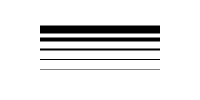Joins:

```from kiva.image import GraphicsContext
from kiva.constants import JOIN_ROUND, JOIN_BEVEL, JOIN_MITER

gc = GraphicsContext((200, 100))
gc.set_line_width(8)

for i, join in enumerate([JOIN_ROUND, JOIN_BEVEL, JOIN_MITER]):
y = 20 + i*20

gc.move_to(y, 80)
gc.line_to(y, y)
gc.line_to(160, y)
gc.set_line_join(join)
gc.stroke_path()

gc.save('joins.png')
```Caps:

```from kiva.image import GraphicsContext
from kiva.constants import CAP_ROUND, CAP_BUTT, CAP_SQUARE

gc = GraphicsContext((200, 100))
gc.set_line_width(8)

for i, cap in enumerate([CAP_ROUND, CAP_BUTT, CAP_SQUARE]):
y = 30 + i*20

gc.move_to(40, y)
gc.line_to(160, y)
gc.set_line_cap(cap)
gc.stroke_path()

gc.save('caps.png')
```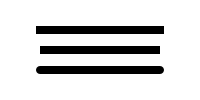Dashes:

```from kiva.image import GraphicsContext

gc = GraphicsContext((200, 100))
dashes = ([6.0, 6.0], [9.0, 3.0], [3.0, 5.0, 9.0, 5.0])
gc.set_line_width(2)

for i, dash in enumerate(dashes):
y = 30.5 + i*20

gc.move_to(40, y)
gc.line_to(160, y)
gc.set_line_dash(dash)
gc.stroke_path()

gc.save('dashes.png')
```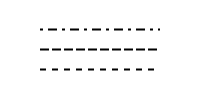Before filling a path, the colour of the fill is via the `set_fill_color()` method, and gradient fills can be done via the `set_linear_gradient()` and `set_radial_gradient()` methods. Finally, there are two different fill modes available: even-odd fill and non-zero winding fill

Winding vs. Even-Odd Fill:

```from numpy import pi
from kiva.image import GraphicsContext
from kiva.constants import FILL, EOF_FILL

gc = GraphicsContext((200, 100))
gc.set_fill_color((0.0, 0.0, 0.0))

gc.move_to(50, 90)
for i in range(1, 6):
theta = 4*pi/5*i
x = 50+40*sin(theta)
y = 50+40*cos(theta)
gc.line_to(x, y)

gc.fill_path()

gc.move_to(150, 90)
for i in range(1, 6):
theta = 4*pi/5*i
x = 150+40*sin(theta)
y = 50+40*cos(theta)
gc.line_to(x, y)

gc.eof_fill_path()

gc.save('fill.png')
```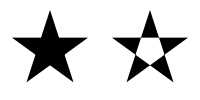### Text¶

Text can be rendered at a point by first setting the font to use, then setting the text location using `set_text_position()` and then `show_text()` to render the text:

```from kiva.fonttools import Font
from kiva.image import GraphicsContext

gc = GraphicsContext((200, 100))

gc.set_font(Font(size=24))
gc.set_text_position(30, 40)
gc.show_text("Hello World")

gc.save('text.png')
```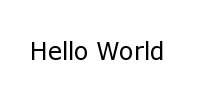Text defaults to being rendered filled, but can be rendered with an outline.

# Kiva Interface Quick Reference¶

This document is a summary of the classes and functions available in Kiva. More specifically, it describes some of the details of the kiva.agg backend, including enumerated types and helper classes.

## Types¶

### Primitive types¶

The following conventions are used to describe input and output types:

color: rect: Either a 3-tuple or 4-tuple. The represented color depends on the graphics context’s pixel format. (origin_x, origin_y, width, height) an int that is 1 or 0 an array/sequence of length-2 arrays, e.g. ((x,y), (x2,y2),...) an array/sequence of rects ((x,y,w,h), (x2,y2,w2,h2), ...) an array/sequence of color stops ((offset,r,g,b,a), (offset2,r2,g2,b2,a2), ...) where offset is some number between 0 and 1 inclusive and the entries are sorted from lowest offset to highest.

### AffineMatrix¶

All of the following member functions modify the instance on which they are called:

__init__(v0, v1, v2, v3, v4, v5):
also __init__()
reset():Sets this matrix to the identity
multiply(AffineMatrix):
multiples this matrix by another
invert():sets this matrix to the inverse of itself
flip_x():mirrors around X
flip_y():mirrors around Y

The rest of the member functions return information about the matrix.

scale() -> float:
returns the average scale of this matrix
determinant() -> float:
returns the determinant

The following factory methods are available in the top-level “agg” namespace to create specific kinds of `AffineMatrix` instances:

translation_matrix(float x, float x)

rotation_matrix(float angle_in_radians)

scaling_matrix(float x_scale, float y_scale)

skewing_matrix(float x_shear, float y_shear)

### FontType¶

__init__(name, size=12, family=0, style=0):
constructs a `FontType` instance
is_loaded() -> bool:
returns True if a font was actually loaded

### CompiledPath¶

Interface is the same as the Path functions in Graphics Context.

### Enumerations¶

The following enumerations are represented by top-level constants in the “agg” namespace. They are fundamentally integers. Some of them also have dicts that map between their names and integer values

line_cap: CAP_BUTT, CAP_ROUND, CAP_SQUARE JOIN_ROUND, JOIN_BEVEL, JOIN_MITER FILL, EOF_FILL, STROKE, FILL_STROKE, EOF_FILL_STROKE NORMAL, BOLD, ITALIC TEXT_FILL, TEXT_INVISIBLE (currently unused) (NOTE: the strings in the dicts omit the `pix_format_` prefix)
• dicts: pix_format_string_map, pix_format_enum_map

• values: pix_format_gray8, pix_format_rgb555, pix_format_rgb565,

pix_format_rgb24, pix_format_bgr24, pix_format_rgba32, pix_format_argb32, pix_format_abgr32, pix_format_bgra32

• dicts: interp_enum_map, interp_string_map

• values: nearest, bilinear, bicubic, spline16, spline36, sinc64, sinc144,

sinc256, blackman64, blackman100, blackman256

marker: (NOTE: the strings in the dicts omit the `marker_` prefix)
• dicts: marker_string_map, marker_enum_map

• values: marker_circle, marker_cross, marker_crossed_circle, marker_dash,

marker_diamond, marker_dot, marker_four_rays, marker_pixel, marker_semiellipse_down, marker_semiellipse_left, marker_x, marker_semiellipse_right, marker_semiellipse_up, marker_square, marker_triangle_down, marker_triangle_left, marker_triangle_right, marker_triangle_up

path_cmd and path_flags are low-level Agg path attributes. See the Agg documentation for more information about them. We just pass them through in Kiva.

path_cmd: path_cmd_curve3, path_cmd_curve4, path_cmd_end_poly, path_cmd_line_to, path_cmd_mask, path_cmd_move_to, path_cmd_stop path_flags, path_flags_ccw, path_flags_close, path_flags_cw, path_flags_mask, path_flags_none

## Graphics Context¶

### Construction¶

__init__(size, pix_format):
Size is a tuple (width, height), pix_format is a string.

### State functions¶

save_state(): array is an even-length tuple of floats that represents the width of each dash and gap in the dash pattern. spread_method is one of the following strings: pad, reflect, repeat. units is one of the following strings: userSpaceOnUse, objectBoundingBox. This method modifies the current fill pattern. same arguments as linear gradient. The direction of the gradient is from the focus point to the center point.

### Clipping functions¶

clip_to_rect(rect):

clip_to_rects(rect_array):

clip():clips using the current path
even_odd_clip():
modifies the current clipping path using the even-odd rule to calculate the intersection of the current path and the current clipping path.

### Path functions¶

begin_path(): returns a blank `CompiledPath` instance draws a cubic bezier curve with control points (x1,y1) and (x2,y2) that ends at point (end_x, end_y) draws a quadratic bezier curve from the current point using control point (cp_x, cp_y) and ending at (end_x, end_y) draws a circular arc of the given radius, centered at (x,y) with angular span as indicated. Angles are measured counter-clockwise from the positive X axis. If “cw” is true, then the arc is swept from the end_angle back to the start_angle (it does not change the sense in which the angles are measured). Sweeps a circular arc from the pen position to a point on the line from (x1,y1) to (x2,y2). The arc is tangent to the line from the current pen position to (x1,y1), and it is also tangent to the line from (x1,y1) to (x2,y2). (x1,y1) is the imaginary intersection point of the two lines tangent to the arc at the current point and at (x2,y2). If the tangent point on the line from the current pen position to (x1,y1) is not equal to the current pen position, a line is drawn to it. Depending on the supplied radius, the tangent point on the line fron (x1,y1) to (x2,y2) may or may not be (x2,y2). In either case, the arc is drawn to the point of tangency, which is also the new pen position. Consider the common case of rounding a rectangle’s upper left corner. Let “r” be the radius of rounding. Let the current pen position be (x_left + r, y_top). Then (x2,y2) would be (x_left, y_top - radius), and (x1,y1) would be (x_left, y_top).

### Drawing functions¶

stroke_path(): if rect is defined, then img is scaled and drawn into it. Otherwise, img is overlaid exactly on top of this graphics context

### Text functions¶

set_text_drawing_mode(text_draw_mode):

set_text_matrix(AffineMatrix):

get_text_matrix() -> AffineMatrix:

set_text_position(float x, float x):

get_text_position() -> (x, y):

show_text(string):

show_text_translate(string, float y, float y):

get_text_extent(string) -> (x,y,w,h):

get_full_text_extent(string) -> (w,h,x,y):
deprecated. Order has been changed for backwards-compatibility with existing Enable.
select_font(name, size, style):

set_font(FontType):

get_font() -> FontType:

set_font_size(int):

set_character_spacing():

get_character_spacing():

set_text_drawing_mode():

show_text_at_point():

### Misc functions¶

width() -> int: height() -> int: Force all pending drawing operations to be rendered immediately. This only makes sense in window contexts, ie- the Mac Quartz backend. A deferred version of flush(). Also only relevant in window contexts. Clears a rect. Not available in PDF context. Save the GraphicsContext to a file. Output files are always saved in RGB or RGBA format; if this GC is not in one of these formats, it is automatically converted. If filename includes an extension, the image format is inferred from it. file_format is only required if the format can’t be inferred from the filename (e.g. if you wanted to save a PNG file as a .dat or .bin). pil_options is a dict of format-specific options that are passed down to the PIL image file writer. If a writer doesn’t recognize an option, it is silently ignored. If the image has an alpha channel and the specified output file format does not support alpha, the image is saved in rgb24 format.

### Functions that are currently stubbed out or not implemented¶

show_glyphs_at_point():